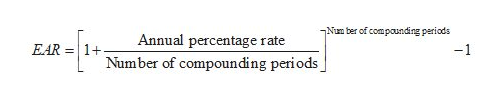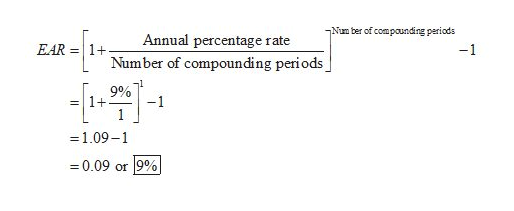# A bank's loan rate for a machine is 9%. Determine the effective rate on the basis of the compounding period for each rate (assuming compound period is annual).

Question
365 views

A bank's loan rate for a machine is 9%. Determine the effective rate on the basis of the compounding period for each rate (assuming compound period is annual).

check_circle

Step 1

The effective interest rate or the effective annual rate (EAR) is the actual rate paid or earned on the loan, an investment, and other financial instruments based on the compounding during the maturity. It is calculated by using the following formula.help_outlineImage TranscriptioncloseNum ber of compounding periods Annual percentage rate EAR 1+ -1 Number of compounding periods fullscreen
Step 2

Calculate the effective ann...help_outlineImage TranscriptioncloseNum ber of compounding periods Annual percentage rate EAR 1+ -1 Number of compoun ding periods -1 9% 1+ -1 1 = -1.09-1 =0.09 or 9% fullscreen

### Want to see the full answer?

See Solution

#### Want to see this answer and more?

Solutions are written by subject experts who are available 24/7. Questions are typically answered within 1 hour.*

See Solution
*Response times may vary by subject and question.
Tagged in